Design and Analyze High Pass Filter using pcbComponent

This example shows how to design and analyze high pass filter. A microstrip high pass filter is designed based on a three-pole (n = 3) Chebyshev high pass prototype with 0.1 dB passband ripple and cut-off frequency ${f}_{c}$ = 1.5 GHz.

Design and Analyze High Pass Filter

The element values of the corresponding low pass Chebyshev prototype are ${g}_{0}$ = ${g}_{4}$ = 1.0, ${g}_{1}$ = ${g}_{3}$ = 1.0316, and ${g}_{2}$ = 1.1474. Using the design equations given in reference  for n = 3 and ${Z}_{0}$ = 50 ohm terminations, we can obtain quasi-lumped circuit elements values as ${C}_{1}$ = ${C}_{3}$ = 2.0571 pF, ${L}_{2}$ =4.6236 nH. A schematic diagram of such a high pass filter taken from reference  representing various feature dimensions is shown below. It is seen here that the series capacitors ${C}_{1}$ and ${C}_{3}$ are realized by identical interdigital capacitors, while the shunt inductor ${L}_{2}$ is realized by a short-circuited stub. A commercial substrate (RT/D 5880) having a relative dielectric constant of 2.2 and thickness of 1.57 mm is chosen for this microstrip filter realization. The dimensions of the interdigital capacitors, such as the finger length, width, spacing between fingers, and number of the fingers are given in the reference .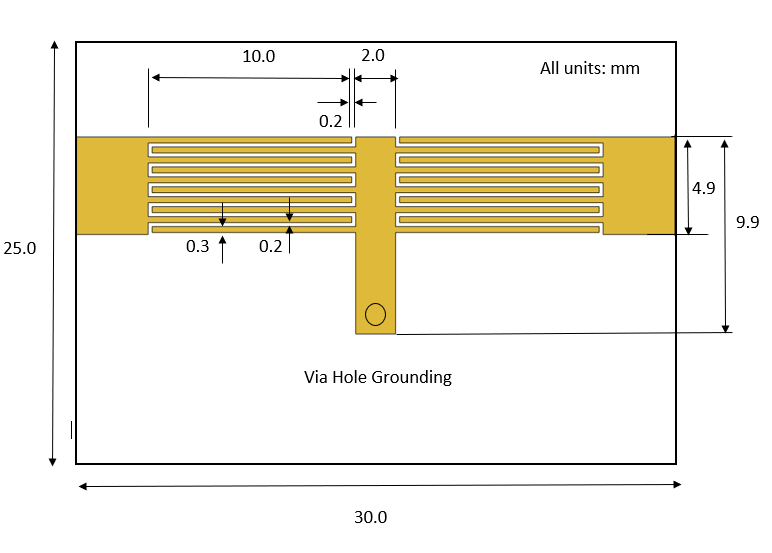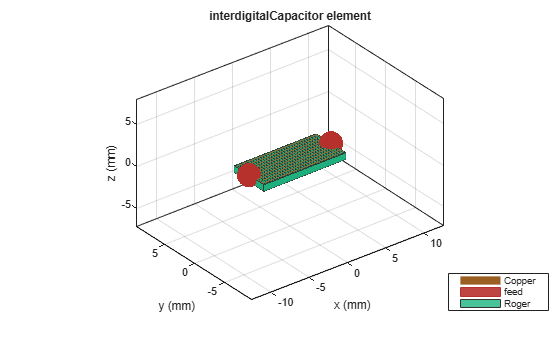Use the interdigitalCapacitor object and change its properties as per given values in reference  to create interdigited fingers. Visualize created object using show

idc = interdigitalCapacitor;
idc.NumFingers = 10;
idc.FingerLength = 10e-3;
idc.FingerWidth = 0.3e-3;
idc.FingerSpacing = 0.2e-3;
idc.FingerEdgeGap = 0.2e-3;
idc.GroundPlaneWidth = 5e-3;
idc.TerminalStripWidth = 0.1e-3;
idc.PortLineLength = 0.1e-3;
idc.PortLineWidth = 4.8e-3;
figure;
show(idc);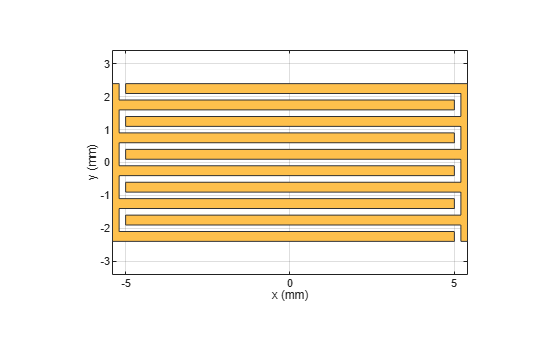Use the pcbComponent on idc object to create a capacitor cap1. Visualize cap1 using show

pcb = pcbComponent(idc);
cap1 = pcb.Layers{1};
figure;
show(cap1);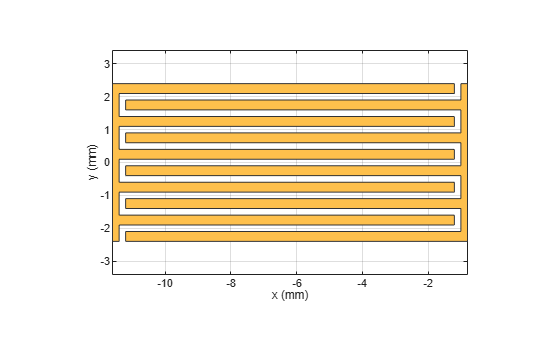Use the copy, rotateZ, rotateX and translate operation methods on capacitor cap1 object to create capacior cap2. Visualize cap2 using show

cap2 = copy(cap1);
cap2 = rotateZ(cap2,180);
cap2 = rotateX(cap2,180);
cap2 = translate(cap2,[-6.2e-3 0 0]);
cap1 = translate(cap1,[6.2e-3 0 0]);
figure;
show(cap2);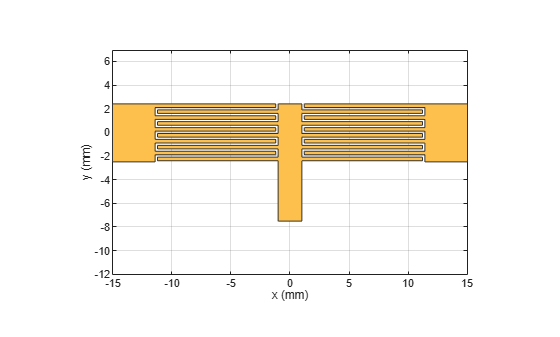Use the traceRectangular object to create both feeding ports port1, port2 and short circuited stub centerArm. Perform a Boolean add operation for the shapes port1, cap2, centerArm, cap1 and port2 to create filter. Visualize filter using show

portW = 4.9e-3;
portL = 3.6e-3;
centerL = 2e-3;
centerW = 9.9e-3;
port1 = traceRectangular("Length",portL,"Width",portW,"Center",[-11.4e-3-portL/2 -0.05e-3]);
port2 = traceRectangular("Length",portL,"Width",portW,"Center",[11.4e-3+portL/2 -0.05e-3]);

centerArm = traceRectangular("Length",centerL,"Width",centerW,"Center",[0 -2.55e-3]);
filter = port1 + cap2 + centerArm + cap1 + port2;
figure;
show(filter);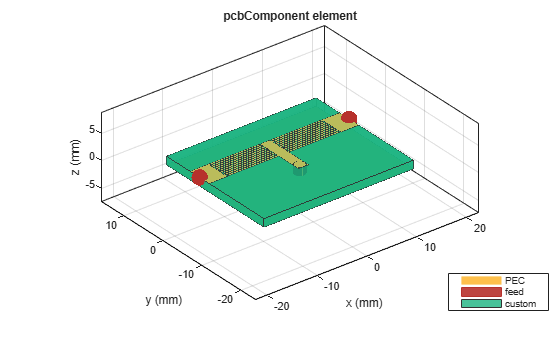Define the substrate parameters and create a dielectric to use in the pcbComponent of the designed filter. Create a groundplane using the traceRectangular shape.

Use the pcbComponent to create a filter PCB. Assign the dielectric and ground plane to the Layers property on pcbComponent. Assign the FeedLocations to the edge of the feed ports. Assign ViaLocations at the edge of stub centerArm. Set the BoardThickness to 1.57 mm on the pcbComponent and visualize the filter. The below code performs these operations and creates the filter pcb object.

substrate = dielectric("EpsilonR",2.2,"LossTangent",0.0009,...
"Name","custom","Thickness",1.57e-3);
gndL = 30e-3;
gndW = 25e-3;
ground = traceRectangular("Length",gndL,"Width",gndW,...
"Center",[0,-4e-3]);

pcb = pcbComponent;
pcb.BoardShape = ground;
pcb.BoardThickness = 1.57e-3;
pcb.Layers ={filter,substrate,ground};
pcb.FeedDiameter = portW/2;
pcb.FeedLocations = [-gndL/2 0 1 3;gndL/2 0 1 3];
pcb.ViaLocations = [0 -6.5e-3 1 3];
figure;
show(pcb);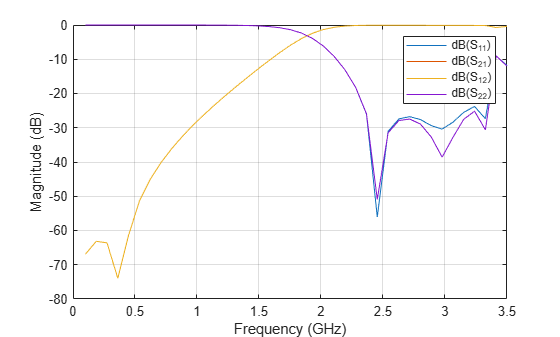Use the sparameters method to calculate the S-parameters for the band stop filter and plot it using the rfplot function.

spar = sparameters(pcb,linspace(0.1e9,3.5e9,40));
figure;
rfplot(spar);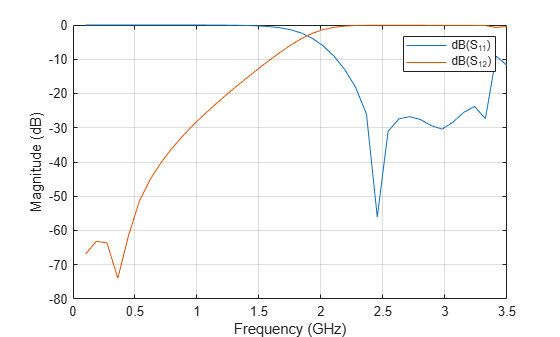As there are four curves in the result, let us analyze the results.

Plot S-Parameters

Analyze the values of ${S}_{11}$, and ${S}_{12}$ to understand the behavior of high pass filter.

figure;
rfplot(spar,1,1);
hold on;
rfplot(spar,1,2);
hold on;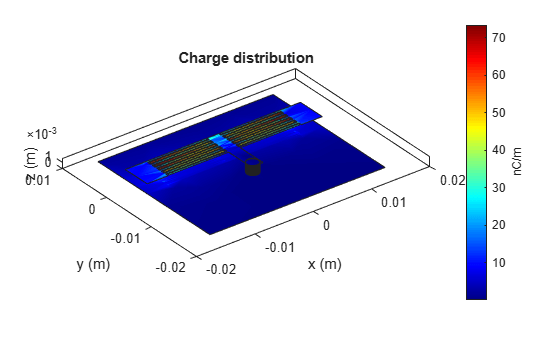The result shows that the filter has -3 dB cut-off frequency ${f}_{c}$ = 1.8 GHz . The ${S}_{12}$ values are greater than -3 dB and ${S}_{11}$ values are less than -10 dB above -3 dB cut-off frequency 1.8 GHz. The designed filter therefore has high pass response and em simulated results matches closely with reference. The 0.3 GHz shift in -3 dB cut-off frequency is observed.

Use the charge method to visualize the charge distribution on the metal surface and dielectric of high pass filter

figure;
charge(pcb,3e9);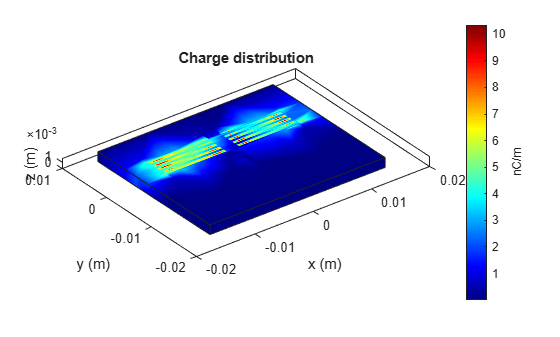figure;
charge(pcb,3e9,'dielectric');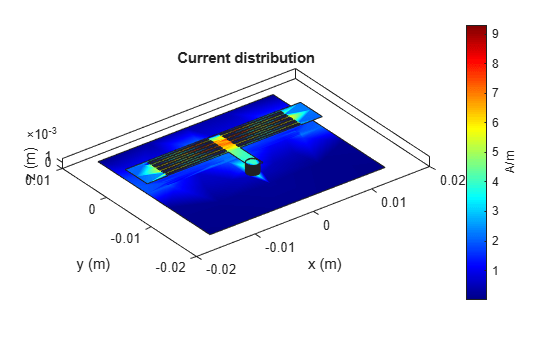Use the current method to visualize the current distribution on the metal surface and volume polarization currents on dielectric of high pass filter

figure;
current(pcb,3e9);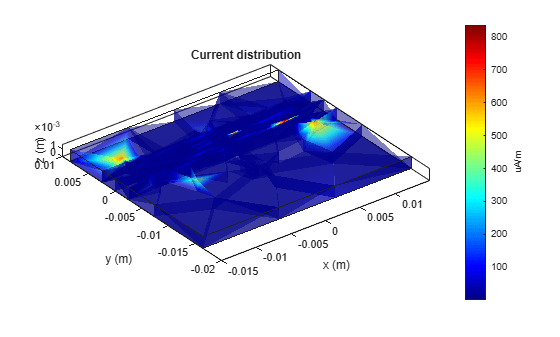figure;
current(pcb,3e9,'dielectric');References

 Jia-Sheng Hong "Microstrip Filters for RF/Microwave Applications", p. 184, John Wiley & Sons, 2nd Edition, 2011.

RF PCB Toolbox Documentation